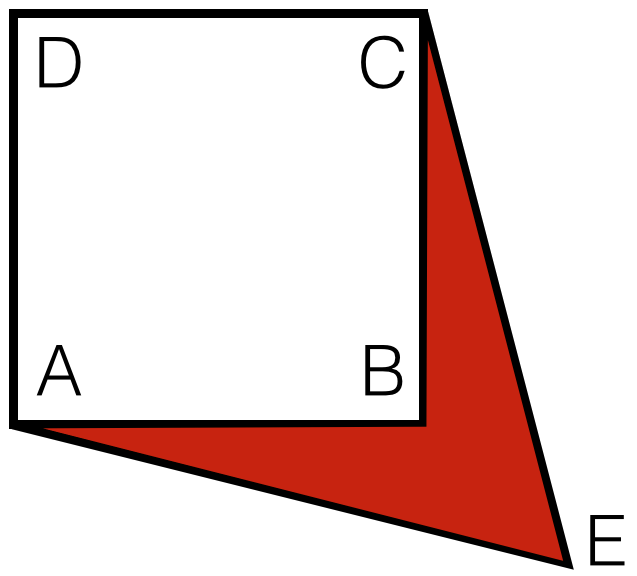# Perfect Polygons

Geometry Level 2The diagram above shows a square $ABCD$ with diagonal $2\text{ cm}$ long, and $AEC$ is an equilateral triangle. Find the area of the quadrilateral $AECB$ in $\text{cm}^2$.

×